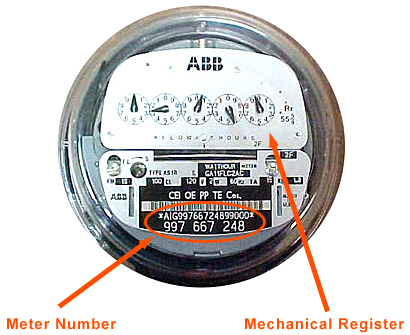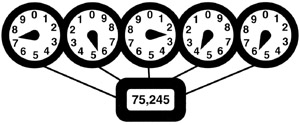icon for My Account
My Account

Most of our customers have a standard electric meter. However, those customers taking advantage of our load management option have a different meter that measures peak demand.

If you have an electronic meter with a digital LCD display, skip directly to the section on electronic load meters.

This is an example of a 9-digit meter number. Depending on the type of meter on your dwelling, your meter number may be 6 to 9 digits in length. Should you have a problem determining what is your correct meter number, write down the number and send us an e-mail at the contact us or call a Customer Service Representative. If you have a standard electric meter with NO load meter, do the following:Stand directly in front of your electric meter, with the meter at eye level. You will notice a series of round dials on the meter face. Each dial has ten numbers (0 - 9) and a pointer like a clock hand. The pointers advance when electricity flows through the meter, so the dials indicate the total number of kilowatt-hours (KWH) you have used.

To determine your correct meter reading, simply read the dials in order from right to left. As you read each dial, write down the number. In most cases, the pointer will be between two numbers on the dial. THE CORRECT NUMBER IS THE LOWER OF THE TWO, EXCEPT WHEN BETWEEN THE 0 AND 9, IN THAT CASE YOU WOULD READ THE 9.When reading each dial, pay attention to which direction it runs - some dials run clockwise, and others run counterclockwise. If you are not careful, this could lead you to misread the dials. Try reading the example above. It should be read as 75,245 KWH.When a pointer rests directly on a number, as in the fourth dial from the right in this example, be sure to check the dial to the immediate right. If that pointer has not passed zero, as shown here, the number in question has not yet been reached. Therefore, the dial above should be read as five, not six. The correct reading for this meter is 75,933 KWH